# How to Convert Decimal Degrees to Degrees Minutes Seconds in Excel

Minutes and seconds are more basic units for measuring rotation than degrees. Using minutes when measuring rotation helps us analyse the values more accurately and efficiently. We can convert decimal degrees into degrees, minutes, and seconds using the VBA application, as it can’t be completed directly in Excel. This tutorial will help you understand how we can convert decimal degrees to degrees, minutes, and seconds in Excel. Here we will demonstrate two methods for converting decimal degrees to degrees, minutes, and seconds and degrees, minutes, and seconds to decimal degrees.

## Convert Decimal Degrees to Degrees Minutes Seconds

Here, we will first create a VBA module and then run it to complete our task. Let us see a simple process to know how we can convert decimal degrees to degrees, minutes, and seconds using a VBA application in Excel.

### Step 1

Consider an Excel sheet with data that is similar to the data shown in the image below.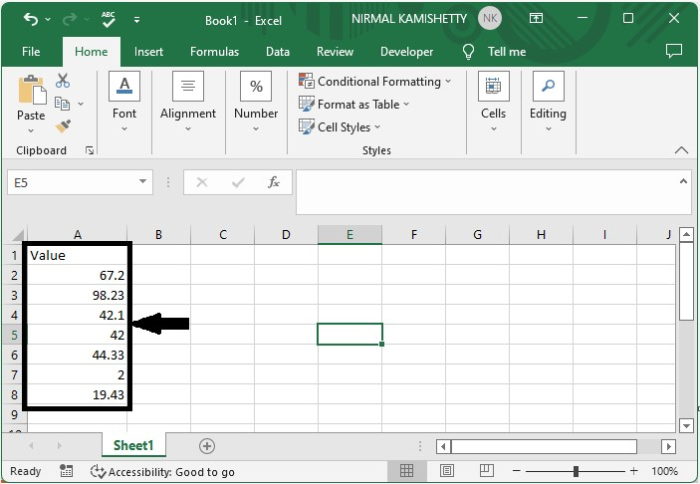Now click right-click on the sheet name and select view code to open the VBA application, then click on inset and select module.

Right click > view code > insert > module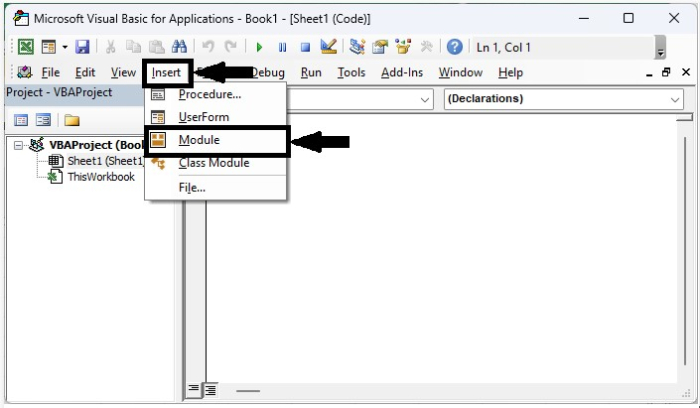### Step 2

Then, as shown in the image below, type program 1 into the text box.

### Example

Sub ConvertDegree()
'Updated By Nirmal
Dim Rng As Range
Dim WorkRng As Range
On Error Resume Next
xTitleId = "Select the range"
Set WorkRng = Application.Selection
Set WorkRng = Application.InputBox("Range", xTitleId, WorkRng.Address, Type:=8)
For Each Rng In WorkRng
num1 = Rng.Value
num2 = (num1 - Int(num1)) * 60
num3 = Format((num2 - Int(num2)) * 60, "00")
Rng.Value = Int(num1) & "°" & Int(num2) & "'" & Int(num3) & "''"
Next
End Sub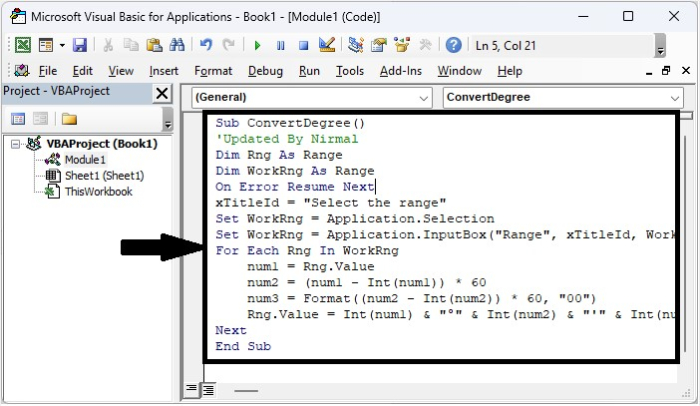### Step 3

Then save the sheet as a macro-enabled template, and then click on F5 to run the macro, select the range you want to covert, and click OK to complete the process.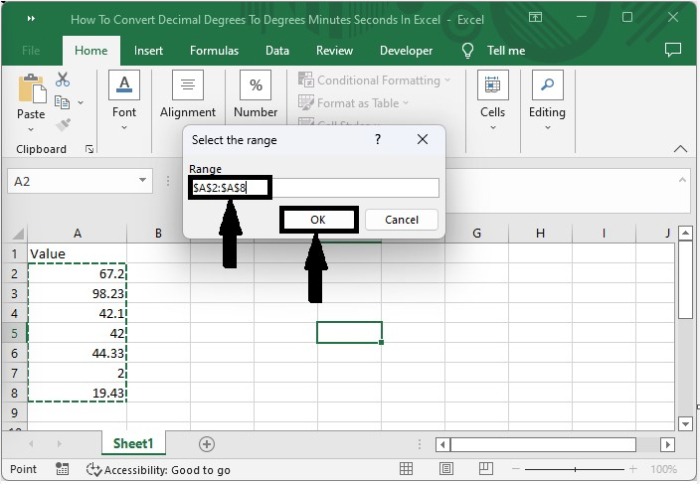## Convert Decimal Degrees Minutes Seconds to Degree

Here, we will first use VBA to create a module and then run it to complete our task. Let us look at a simple procedure for converting decimal degree minutes to degrees.

### Step 1

Let us consider a new Excel sheet and right-click on the sheet name and select view code to open a VBA application.

Then click on insert, select module, and enter program 2 in the text box as shown in the below image.

### Example

Function ConvertDecimal(pInput As String) As Double
'Update by nirmal
Dim xDeg As Double
Dim xMin As Double
Dim xSec As Double
xDeg = Val(Left(pInput, InStr(1, pInput, "°") - 1))
xMin = Val(Mid(pInput, InStr(1, pInput, "°") + 2, _InStr(1, pInput, "'") - InStr(1, pInput, _
"°") - 2)) / 60
xSec = Val(Mid(pInput, InStr(1, pInput, "'") + _
2, Len(pInput) - InStr(1, pInput, "'") - 2)) _
/ 3600
ConvertDecimal = xDeg + xMin + xSec
End Function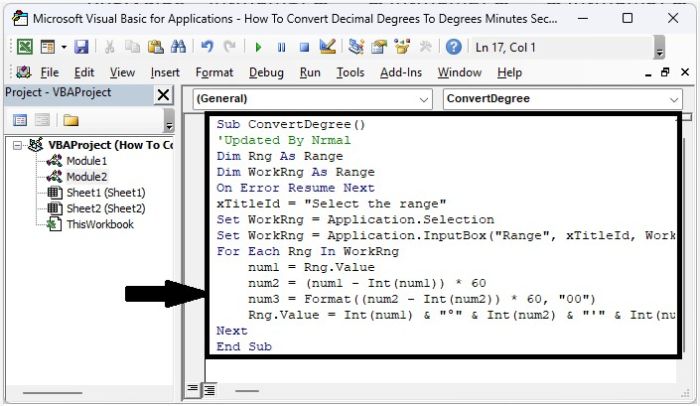### Step 2

Now save the sheet, and in the cell, enter the formula as =ConvertDecimal("10° 27' 36""") and click OK to get the result shown in the below image.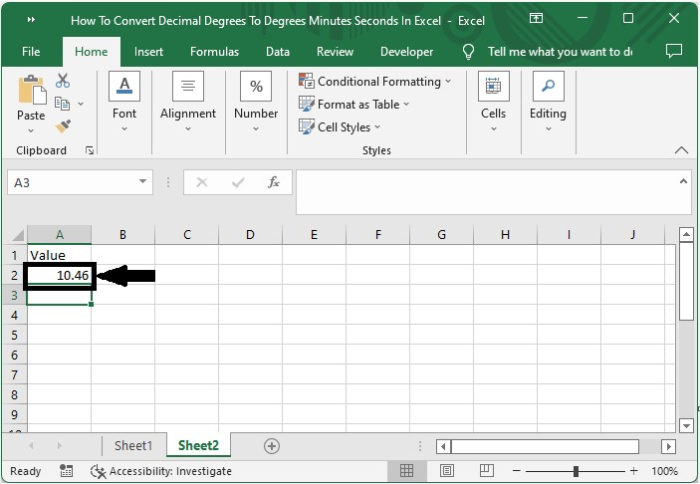## Conclusion

In this tutorial, we used a simple example to demonstrate how you can convert decimal degrees to degree minutes in Excel.

Updated on: 06-Feb-2023

4K+ Views